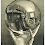## Tuesday, December 23, 2008

### Vacation From School

In how many ways can you arrange 100 identical stones into 3 piles so that each pile has at least 1 stone in it.

1.I'll take a crack at it; Let me see:

If the piles are non-identical (indexed with 1,2,3), then the first pile may have n = between 1 and 98 stones. In each of these cases, the number of stones in the second pile can range from 1 to (100 - (n + 1)), and after that the distribution is uniquely identified. So the total number of combinations is SUM(n=1 to 98)[99-n], or SUM(1,2,3,...,98), which is equal to 4,581.

If the piles are identical (so that, for instance, {1,1,98} is the same distribution as {1,98,1}), we can group the combinations above and count the number of such groups, leaving out the "repeated" combinations. There is no {n,n,n} combination, since 100 % 3 <> 0. There are 49 {n,n,m} combinations (one for each number such that m = 100 - 2*n > 0), and each occurs 3 times ([n,n,m],[n,m,n],[m,n,n]). The remaining 4,434 (4,581 - 49*3) combinations are [n,m,l] combinations with no repeated entries, and they occur 6 times each ([n,m,l],[n,l,m],[m,n,l],[m,l,n],[l,m,n],[l,n,m]), for a total of 739 unique combinations. Now, 49 + 739 = 788; so I believe that is our answer?

2.98+97+96+95+......+3+2+1 = 4851

3.Nice work, both of you. Here's another way to explain it, although oudeis did a great job above.

By removing one stone from each pile, this is the number of ways you can arrange m-k identical stones into k (possibly empty) piles.

Now, view the k piles as a numbered set .
Write on each stone the number of a chosen pile and order the stones accordingly.

The numbered stones constitute a combination with repetition of k elements (the numbers) choose m-k (the stones). This can be done in

C'(k,m-k) = C(m-1,m-k) =

(m - 1)!
----------------- ways.
(m - k)!(k - 1)!

Leave your answer or, if you want to post a question of your own, send me an e-mail. Look in the about section to find my e-mail address. If it's new, I'll post it soon.

Please don't leave spam or 'Awesome blog, come visit mine' messages. I'll delete them soon after.

Enter your Email and join hundreds of others who get their Question of the Day sent right to their mailbox

The Lamplight Manor Puzz 3-D